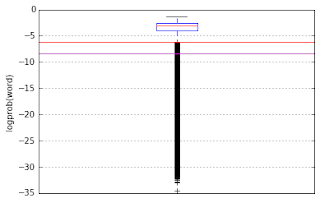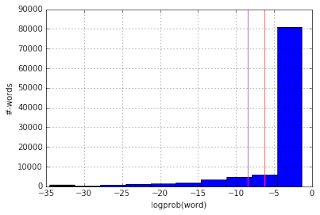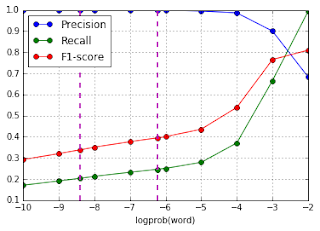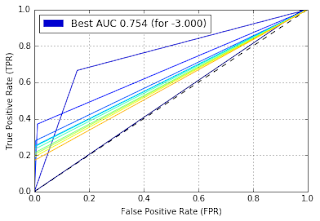## Saturday, March 26, 2016

### Introduction

The idea for this post came about based on a talk I attended last week. In that talk, the presenter described problems with detecting corruption in OCR text. This post describes a possible solution to the problem. The idea for the solution is based on the intuition that sequences of characters in corrupted regions of the text would be relatively uncommon compared to the uncorrupted text. This is because the uncorrupted text would be made up of words which are repeated over and over in different documents and even within the same document. This would not be true for corrupted words, since errors tend to be non-systemic. In some respects, this is similar to the intuition behind the Lucene Spellchecker.

My solution consists of building a unigram (and later trigram) character language model out of our documents, and from that build up a table of transition probabilities. Using this model, I then compute the generation probability of any new word with respect to this language model. The generation probability is just the product of the individual transition probabilities of the unigrams (or trigrams) of this word. For ease of computation, I consider the sum of log probabilities, which is the same thing. To make the log probability comparable across different word sizes, I normalize it by the length of the word.

If we look at enough words, we end up with a univariate distribution of normalized generation probabilities (or log probabilities). If we think of an corrupted word as an anomaly in this distribution, detecting corrupted words becomes just a matter of finding an appropriate threshold that splits the distribution into normal and outlier.

### Data Preprocessing

For my data, I used the RETAS OCR Evaluation Dataset. The RETAS (REcursive Text Alignment Scheme) dataset consists of the OCR output of 160 scanned fiction books (100 English, 20 French, 20 German and 20 Spanish) downloaded from the Internet Archive, with the ground truth represented by the corresponding book from Project Gutenberg.

For this test, I will consider only the English books. The 100 English books (contained in the IA_texts/eng subdirectory of the RETAS dataset) are organized in 20 volumes in Project Gutenberg (contained in the GUT_texts/eng subdirectory). A file eng_pairs.txt defines the mapping between the files in IA_texts/eng and GUT_texts/eng. For convenience, I concatenate the OCR files so they correspond to the Gutenberg volumes into a new directory OCR_texts/eng. The code to do so is shown below:

 ```1 2 3 4 5 6 7 8 9 10 11 12 13 14 15 16 17 18 19 20 21 22 23 24 25 26 27``` ```# Source: src/preprocess.py # -*- coding: utf-8 -*- import os # Scan pairs file and associate OCR files with Gutenberg file fpairs = open("../data/eng_pairs.txt", 'rb') io_pairs = {} for line in fpairs: ocr_name, gut_name = line.strip().split('\t') if io_pairs.has_key(gut_name): io_pairs[gut_name].append(ocr_name) else: io_pairs[gut_name] = [ocr_name] fpairs.close() # Write out consolidated OCR file for each Gutenberg file gutenberg_dir = "../data/GUT_texts/eng" ia_dir = "../data/IA_texts/eng" ocr_dir = "../data/OCR_texts/eng" for gut, ias in io_pairs.items(): focr = open(os.path.join(ocr_dir, gut + ".txt"), 'wb') for ia in ias: fia = open(os.path.join(ia_dir, ia + ".txt"), 'rb') for line in fia: focr.write(line) fia.close() focr.close() ```

### Training

Next I split the files 75/25 into a training and an evaluation set, ie, I use 15 of the 20 files for training and the rest for evaluation. In the training phase I build up the matrix of transition probabilities, and in the evaluation set, I use these transition probabilities to compute normalized word generation log probabilities and build up the distribution.

Important thing to note here is that I use the ground truth (Gutenberg) files in the training / model building phase, since I am interested in the true transition probabilities given (a large sample of) the corpus. On the other hand, when I evaluate, I compute the normalized log probabilities for words from the OCR files, since I am interested in finding words that have anomalous word generation probabilities.

Also, my model of transition probabilities is necessarily incomplete, since it is built from a sample of the words in the English language. Words in the evaluation set with transitions that don't exist in the model would result in a generation probability of 0. To prevent this, I apply Laplace smoothing and provide a small probability mass of 1e-15 for transitions that have never seen before.

The code shown below builds a unigram character model and then computes the word probabilities for all distinct words in the evaluation set.

 ```1 2 3 4 5 6 7 8 9 10 11 12 13 14 15 16 17 18 19 20 21 22 23 24 25 26 27 28 29 30 31 32 33 34 35 36 37 38 39 40 41 42 43 44 45 46 47 48 49 50 51 52 53 54 55 56 57 58 59 60 61 62 63 64 65 66 67 68 69 70 71 72 73 74 75 76 77 78 79 80 81 82 83 84 85 86 87 88 89 90 91 92 93 94 95 96 97 98 99 100 101 102 103``` ```# Source: src/build_model.py # -*- coding: utf-8 -*- from sklearn.cross_validation import train_test_split import nltk import math import os TRAIN_DIR = "../data/GUT_texts/eng" TEST_DIR = "../data/OCR_texts/eng" SMOOTHING_PARAM = 1e-15 LOG_SMOOTHING_PARAM = math.log(SMOOTHING_PARAM) def char_transitions(word): """ Converts a word into a list of character transitions. For example: "alice" will be converted to: [" :a", "a:l", "l:i", "i:c", "c:e", "e: "] """ transitions = [] word_chars = [c for c in " " + word + " "] for char_pair in nltk.bigrams(word_chars): transitions.append(char_pair) return transitions def build_model(train_files): """ Builds a character transition probability matrix from training data. Probabilities are smoothed to account for unseen transitions, and probabilities are returned as logarithms for computational efficiency reasons. """ trans_probs = {} print("Building model...") for train_file in train_files: print(" reading %s" % (train_file)) ftrain = open(os.path.join(TRAIN_DIR, train_file), 'rb') for line in ftrain: line = line.strip().lower().decode("latin-1") words = nltk.word_tokenize(line) for word in words: for pair in char_transitions(word): if trans_probs.has_key(pair): prob_values = trans_probs[pair] if prob_values.has_key(pair): trans_probs[pair][pair] += 1 else: trans_probs[pair][pair] = 1 else: trans_probs[pair] = {pair: 1} ftrain.close() # Normalize each left => right transitions to sum to 1 (with smoothing) log_trans_probs = {} for left, rights in trans_probs.items(): counts = sum([v for k, v in rights.items()]) norm_rights = {k: math.log(1.0 * v / (counts + (SMOOTHING_PARAM * len(rights)))) for k, v in rights.items()} log_trans_probs[left] = norm_rights return log_trans_probs def compute_word_logprob(char_pairs, model): """ Returns the log probability of the word being generated as the sum of log probabilities of individual character transitions, normalized by the length. """ if len(char_pairs) == 0: return LOG_SMOOTHING_PARAM logprob_word = 0.0 for char_pair in char_pairs: try: logprob_word += model[char_pair][char_pair] except KeyError: logprob_word += LOG_SMOOTHING_PARAM return logprob_word / len(char_pairs) def compute_word_probabilities(test_file, model): """ Compute word log probabilities for all unique words in specified test file. """ word_probs = {} print("Computing word log-probabilities for %s" % (test_file)) ftest = open(os.path.join(TEST_DIR, test_file), 'rb') for line in ftest: line = line.strip().lower().decode("latin-1") words = nltk.word_tokenize(line) for word in words: if word_probs.has_key(word): continue pairs = char_transitions(word) word_prob = compute_word_logprob(pairs, model) word_probs[word] = word_prob ftest.close() return word_probs ############################## main ################################ files = os.listdir("../data/GUT_texts/eng") train_files, test_files = train_test_split(files, test_size=0.25, random_state=42) model = build_model(train_files) fprobs = open("../data/word_probs.txt", 'wb') for test_file in test_files: word_logprobs = compute_word_probabilities(test_file, model) for k, v in word_logprobs.items(): fprobs.write("%s\t%s\t%.7f\n" % (test_file, k.encode("latin-1"), v)) fprobs.close() ```

### Evaluation

The code above will write out a file that looks like this. The first column is the name of the OCR file (generated from the data preprocessing step) that contains the word, the second column is the word itself, and the last column is the normalized word probability for generating the word, computed as the sum of the log probabilities of individual transitions divided by the length of the word.

 ```1 2 3 4 5 6``` ```11.txt fawn -2.9960562 11.txt writings -2.5479031 11.txt pagb -17.2524527 11.txt fig- -3.1643667 11.txt foun -2.1711398 ... ```

If we plot the distribution of log probabilities, we get something that looks like this. Both the box plot and histogram show a very long and heavy tail of outliers. The red and magenta lines running across the boxplot and the histogram indicate the beginning of the outlier and extreme outliers as computed by Tukey's Interquartile Range (IQR) formula - everything below these lines in the box plot and everything to the left of the lines in the histogram are considered outliers according to this formula.Here is the code to plot the charts above. The thresholds computed from Tukey's IQR formula are -6.2291706 and -8.4098755 for outlier and extreme outlier respectively.

 ```1 2 3 4 5 6 7 8 9 10 11 12 13 14 15 16 17 18 19 20 21 22 23 24 25 26 27 28 29 30 31 32 33 34 35 36 37 38 39 40 41 42 43 44 45 46 47 48 49 50 51``` ```# Source: src/viz_distrib.py # -*- coding: utf-8 -*- import matplotlib.pyplot as plt import numpy as np def compute_outlier_thresholds(probs): """ Compute outlier points using median/quartile based IQR approach. IQR = Q75 - Q25 if |x - Q25| > 1.5 IQR => x is outlier if |x - Q25| > 3.0 IQR => x is extreme outlier. """ probs_array = np.array(probs) q25 = np.percentile(probs_array, 25) q75 = np.percentile(probs_array, 75) iqr = q75 - q25 return q25 - (1.5 * iqr), q25 - (3.0 * iqr) def boxplot(probs, o_thresh, eo_thresh): """ Draw boxplot from data. """ plt.boxplot(probs) plt.ylabel("logprob(word)") plt.axhline(y=o_thresh, color='r') plt.axhline(y=eo_thresh, color='m') plt.xticks([]) plt.grid(True) plt.show() def histogram(probs, o_thresh, eo_thresh): """ Draw histogram from data. """ plt.hist(probs, bins=10) plt.grid(True) plt.xlabel("logprob(word)") plt.ylabel("#-words") plt.axvline(x=o_thresh, ymin=0, color='r') plt.axvline(x=eo_thresh, ymin=0, color='m') plt.show() ############################ main ########################### fprobs = open("../data/word_probs.txt", 'rb') probs = [] for line in fprobs: fname, word, prob = line.strip().split('\t') probs.append(float(prob)) fprobs.close() o_thresh, eo_thresh = compute_outlier_thresholds(probs) print("Thresholds: outlier < %.7f, extreme outlier < %.7f" % (o_thresh, eo_thresh)) boxplot(probs, o_thresh, eo_thresh) histogram(probs, o_thresh, eo_thresh) ```

These outlier points are a good starting point for finding a threshold, but not necessarily the best in terms of accurate prediction of corrupted words. In order to experimentally find the optimum threshold, we need to have a way to predict that a word is an outlier and we need some ground truth indicating that it is (or is not) an outlier.

So I choose various thresholds and for each word, and compute if it is an outlier based on if its normalized generation log probability is less than the threshold. This would be the model's "prediction". Whether the word is actually an anomaly or not is determined by whether it is present in the Gutenberg version of the file, which constitutes the "label". With this, I calculate Precision, Recall and F1-score for various threshold settings. I also compute the Reciever Operating Characteristics (ROC) curves for each threshold and compute the Area under the Curve (AUC) to determine the "best" threshold. The code to do so is shown below.

 ```1 2 3 4 5 6 7 8 9 10 11 12 13 14 15 16 17 18 19 20 21 22 23 24 25 26 27 28 29 30 31 32 33 34 35 36 37 38 39 40 41 42 43 44 45 46 47 48 49 50 51 52 53 54 55 56 57 58 59 60 61 62 63 64 65 66 67 68 69 70 71 72 73 74 75 76 77 78 79 80 81 82 83 84 85 86 87 88 89 90 91 92 93 94 95 96 97 98 99 100 101 102 103 104 105 106 107 108 109 110 111 112 113 114 115 116 117 118 119 120 121 122 123 124 125 126 127 128 129 130 131 132 133 134 135 136 137 138 139 140 141 142 143 144 145 146 147 148 149 150 151 152``` ```# Source: src/evaluate_model.py # -*- coding: utf-8 -*- from matplotlib import cm from sklearn.metrics import precision_recall_fscore_support, roc_curve, auc import matplotlib.patches as mpp import matplotlib.pyplot as plt import nltk import os import numpy as np O_THRESH = -6.2291706 EO_THRESH = -8.4098755 def build_dictionary(fname): """ Build a dictionary of words from the ground truth file. """ words = set() fdict = open(os.path.join("../data/GUT_texts/eng", fname), 'rb') for line in fdict: line = line.strip().lower().decode("latin-1") for word in nltk.word_tokenize(line): words.add(word) fdict.close() return words def make_predictions(threshold): """ Makes predictions on the words from test set, using the threshold and the word's log probability. Returns two arrays of true labels (computed based on dictionary matching against ground truth from Gutenberg) and predicted labels based on the log probability and specified threshold. We use this to tune our threshold. """ fprobs = open("../data/word_probs.txt", 'rb') y_labels = [] y_preds = [] prev_fname = None valid_words = {} for line in fprobs: line = line.strip().decode("latin-1") fname, word, prob = line.split('\t') if fname != prev_fname: print("Building dictionary for %s" % (fname)) valid_words = build_dictionary(fname) # A predicted corrupted word would have a logprob that # is below the threshold if float(prob) < threshold: y_preds.append(True) else: y_preds.append(False) # A corrupted word would not exist in the ground truth # dictionary if word in valid_words: y_labels.append(False) else: y_labels.append(True) prev_fname = fname fprobs.close() return y_labels, y_preds def chart_precision_recall(): """ Draws a Precision Recall Chart. """ fpr = open("../data/prec_recalls.txt", 'rb') thresh_spl = [] threshs = [] precs = [] recalls = [] f1scores = [] for line in fpr: thresh, prec, recall, f1score = line.strip().split('\t') thresh = float(thresh) if int(thresh) != thresh: thresh_spl.append(thresh) threshs.append(thresh) precs.append(float(prec)) recalls.append(float(recall)) f1scores.append(float(f1score)) fpr.close() plt.plot(threshs, precs, color='b', marker='o', label="Precision") plt.plot(threshs, recalls, color='g', marker='o', label="Recall") plt.plot(threshs, f1scores, color='r', marker='o', label="F1-score") plt.grid(True) plt.xlabel("logprob(word)") plt.legend(loc="best") for thresh in thresh_spl: plt.axvline(x=thresh, color='m', linestyle='--', linewidth=2) plt.show() def compute_roc_scores(y_labels, y_preds): """ Calculates the FPR and TPR for a given run. """ fpr, tpr, _ = roc_curve(y_labels, y_preds) return zip(fpr, tpr) def chart_roc(): """ Draws a ROC Chart. """ i = 0 best_auc = 0.0 best_threshold = None best_color = None colors = [cm.jet(x) for x in np.linspace(0, 1.0, 15)] plt.plot([0, 1], [0, 1], 'k--') frocs = open("../data/roc_scores.txt", 'rb') for line in frocs: threshold, scores = line.strip().split('\t') threshold = float(threshold) tprs = [] fprs = [] for score_pair in scores.split(','): fpr, tpr = [float(x) for x in score_pair.split(' ')] tprs.append(tpr) fprs.append(fpr) roc_auc = auc(fprs, tprs) plt.plot(fprs, tprs, color=colors[i]) if roc_auc > best_auc: best_auc = roc_auc best_threshold = threshold best_color = colors[i] i += 1 frocs.close() plt.grid(True) plt.xlabel("False Positive Rate (FPR)") plt.ylabel("True Positive Rate (TPR)") legend = mpp.Patch(color=best_color, label="Best AUC %.3f (for %.3f)" % (best_auc, best_threshold)) plt.legend(handles=[legend], loc="best") plt.show() ################################# main ############################### fmetrics = open("../data/prec_recalls.txt", 'wb') frocs = open("../data/roc_scores.txt", 'wb') thresholds = [-float(x) for x in range(2, 11)] thresholds.append(O_THRESH) thresholds.append(EO_THRESH) for threshold in sorted(thresholds, reverse=True): print("Computing Precision/Recall at %.5f..." % (threshold)) y_labels, y_preds = make_predictions(threshold) # Precision Recall prfs = precision_recall_fscore_support(y_labels, y_preds, average="binary") fmetrics.write("%.5f\t%.5f\t%.5f\t%.5f\n" % (threshold, prfs, prfs, prfs)) # ROC Curve roc_scores = compute_roc_scores(y_labels, y_preds) frocs.write("%.5f\t%s\n" % (threshold, ",".join([str(x)+" "+str(x) for x in roc_scores]))) fmetrics.close() frocs.close() # Precision Recall Chart for visualization chart_precision_recall() # ROC chart for visualization chart_roc() ```

The resulting Precision-Recall curve and ROC curves are shown below. As can be seen, a good tradeoff between precision and recall can be found at a threshold of -3. Also, the ROC curve for -3 has the best AUC of 0.754.The confusion matrix for the model at this threshold is shown below. As you can see, while the majority (0.722) of the results lie on the diagonal, there are 23,171 (0.23) false positives, ie, the model reports regular words as errors. However, this can be minimized by checking the model prediction against a dictionary of words. Of greater concern is the 5,128 (about 0.05) false negatives, ie, corrupted words that go unreported.

 ```1 2``` ```[[27598 5128] [23171 46089]] ```

Here are some examples of what this model thinks are corrupted text, along with their normalized log probability score.

 ```1 2 3 4 5 6 7 8 9 10 11``` ```pagb -17.252 fig- -3.164 awdry -3.241 jjshe -13.179 said'pig -3.861 -9^4./ -25.933 shai-p -3.862 -ticket -3.003 wood^ -13.571 tfbi^/ -18.152 wjk -12.639 ```

To summarize, here are some key metrics for the unigram character based transition model.

1. Precision: 0.89988
2. Recall: 0.66545
3. F1-score: 0.76511
4. AUC: 0.754
5. Accuracy: 0.722

### Extending the Model

I attempt to improve the performance of the model by giving it a little more context (or memory). Instead of unigram character transitions, I extend the model to compute trigram character transition probabilities. Extending it is simply a matter of replacing the function char_transitions() in src/build_model.py with the following code.

 ```1 2 3 4 5 6 7 8 9 10 11``` ```def char_transitions(word): """ Convert a word into a list of trigram transitions. For example, "alice" will be converted to: [(' al', 'ali'), ('ali', 'lic'), ('lic', 'ice'), ('ice', 'ce ')] """ transitions = [] char_trigrams = ["".join(x) for x in nltk.trigrams([c for c in " " + word + " "])] for trigram_pair in nltk.bigrams(char_trigrams): transitions.append(trigram_pair) return transitions ```

With this, my normalized word generation log probability distribution looks quite different and a lot less spectacular than the unigram version. Notice how the plots report a complete absence of outliers using the IQR formula. However, notice also that the plot is much wider - the ranges here are 10x on a log scale compared to the unigram version.Evaluating this model at various normalized log probability thresholds between 0 to -40 (beyond which there are no words to be found), gives us the following Precision Recall and ROC charts. This time the best tradeoff between Precision and Recall is at the -5 threshold, with a much higher AUC of 0.849.The confusion matrix for this model at the -5 threshold is shown below. The accuracy for this model is 0.834 compared to 0.722 for the previous model. Both False positive (13254; 0.13) and False Negatives (3659; 0.035) are lower than the previous model's 0.23 and 0.05 respectively.

 ```1 2``` ```[[29067 3659] [13254 56006]] ```

Here are some examples of what this model thinks is corrupted text. Norice that it found an additional one "foun" that the unigram model missed.

 ```1 2 3 4 5 6 7 8 9 10 11``` ```pagb -28.538 fig- -14.479 foun -3.051 awdry -9.874 jjshe -25.952 said'pig -20.054 -9^4./ -34.539 shai-p -28.028 -ticket -7.256 wood^ -18.039 tfbi^/ -34.539 ```

Finally, a quick summary of the trigram model's metrics.

1. Precision: 0.96158
2. Recall: 0.76258
3. F1-score: 0.85060
4. AUC: 0.849
5. Accuracy: 0.834

### Conclusion

State of the art OCR software claim accuracy rates of 99.7% and above, but as this article How Good Can It Get? Analysing and Improving OCR Accuracy in Large Scale Historic Newspaper Digitisation Programs by Rose Holley of the Australian Newspaper Digitization Program explains, OCR accuracy depends on document quality and varied between 71% to 98.02% on their document set. An accuracy of 83.4% from my trigram model doesn't sound quite that bad by comparison. Of course, I am talking about slightly different things - the article refers to the accuracy of the OCR software itself, ie had my data been OCR'ed by this particular system, it would have given me data that was between 71-98.02% the same as the input text. My accuracy number refers to how effectively I can find corrupted words in the output of the OCR process.

There are some things I can do to make the model better. I have already mentioned post-processing the model predictions through an English language dictionary to increase the accuracy. Another one could be some rule based filters that look for the presence of punctuation characters in the text. Another possibility might to be to create a better model by cross-validation rather than choosing the first model.

Finally, a big shout-out to the RETAS folks for the dataset. They built the RETAS dataset for their paper - A Fast Alignment Scheme for Automatic OCR Evaluation of Books, by Yalniz and Manmatha, ICDAR'11 to exercise their alignment tool described there. While I didn't use their alignment tool, the RETAS dataset gave me the means to test my idea, so I am very thankful to them for having built it and for sharing it with me.